# 线性表的定义

• 有且仅有一个开始结点，它没有直接前驱，而仅有一个直接后继
• 有且仅有一个终端结点，它没有直接后继，而仅有一个直接前驱
• 其余的内部结点都有且仅有一个直接前驱和一个直接后继

# 线性表的抽象数据类型定义

ADT List{
数据对象：D = {ai|ai属于Elemset,(i = 1,2,...,n,n >= 0)}
数据关系：R = {<ai-1,ai>|ai-1,ai属于D,(i = 2,3,...,n)}
基本操作：
void InitList(&L);			//初始化线性表
void DestroyList(&L);		//销毁线性表
int ListInsert(&L,i,e);		//向线性表插入元素
int ListDelete(&L,i,&e);	//从线性表删除元素
void ClearList(&L);			//清空线性表
int ListEmpty(&L);			//判断线性表是否为空
int ListLength(&L);			//求线性表的长度
int GetElem(&L,i,&e);		//找到线性表指定位置的元素值
int LocateElem(&L,e);		//找到线性表指定元素值的位置
...


# 线性表的顺序表示和实现

1. 顺序存储结构
2. 链式存储结构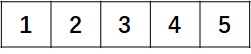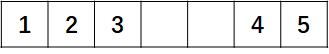## 元素存储位置的计算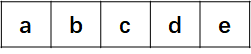LOC(ai+1) = LOC(ai) + x

LOC(ai) = LOC(a1) + (i - 1)x

## 顺序表的实现

• 地址连续
• 依次存放
• 随机存取
• 类型相同

#define MAX_SIZE 10		//线性表初始长度
#define ElemType int
typedef struct{
ElemType data[MAX_SIZE];
int length;		//线性表长度
}SqList;


## 顺序表的初始化

SqList InitList(){
//声明顺序表
SqList l;
l.length = 0;	//初始长度为0
return l;
}


typedef struct{
int *data;		//声明动态数组
int length;		//线性表长度

}SqList;

SqList InitList(){
//声明顺序表
SqList l;
l.data = (int*) malloc(sizeof(int) * MAX_SIZE);
if(l.data == NULL){
exit(-1);
}
l.length = 0;	//初始长度为0
return l;
}


## 顺序表的销毁

void DestroyList(SqList l){
if(l.data){
free(l.data);	//释放动态数组内存
}
l.data = NULL;
}


## 清空顺序表

void ClearList(SqList l){
l.length = 0;	//将顺序表的长度置为0
}


## 求顺序表长度

int GetLength(SqList l){
return l.length;
}


## 判断顺序表是否为空

int ListEmpty(SqList l){
if(l.length == 0){
return 1;
}else{
return 0;
}
}


## 顺序表的插入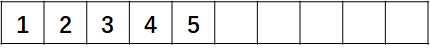1. 表尾插入
2. 非表尾插入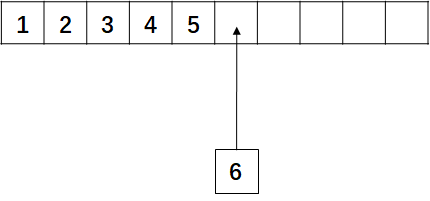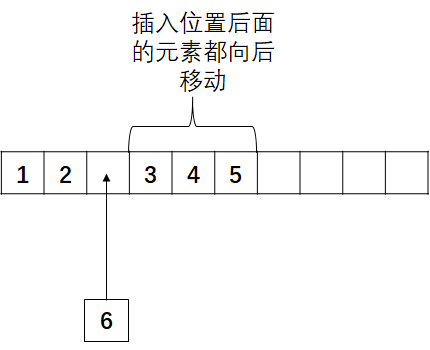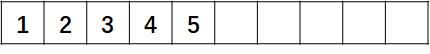1. 判断插入位置pos是否合法
2. 判断顺序表的存储空间是否已满，若已满，返回-1
3. 将pos位置之后的所有元素向后移动一位
4. 将新元素插入到pos位置
5. 顺序表长度length加1，此时插入成功，返回1

int InsertList(SqList *l,int pos,int val){
int i;
//判断插入位置pos是否合法
if(pos < 1 || pos > l->length + 1){
return -1;
}
//判断顺序表是否已满
if(l->length == MAX_SIZE){
return -1;
}
//将pos位置后面的元素向后移动一位
for(i = l->length - 1;i >= pos - 1;i--){
l->data[i + 1] = l->data[i];
}
//将新元素插入到pos位置
l->data[pos - 1] = val;
//顺序表长度加1
l->length++;
return 1;//插入成功
}


• n + 1：这是表尾元素的后一个位置，我们知道这个位置在表未满的情况下是可以插入的，此时插入所需要移动的元素个数为0
• n：这是表尾元素，此时插入所需要移动的元素个数为1，仅移动表尾元素即可
• n - 1：这是倒数第二个元素，此时插入所需要移动的元素个数为2
• 1：依次类推，直到表头元素，此时插入所需要移动的元素个数为n

$\frac{1}{n + 1}(0 + 1 + ... + n - 1 + n) = \frac{1}{n + 1}\frac{n(n + 1)}{2} = \frac{n}{2}$

## 顺序表的删除1. 表尾删除
2. 非表尾删除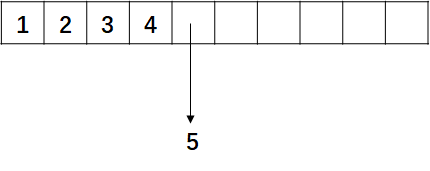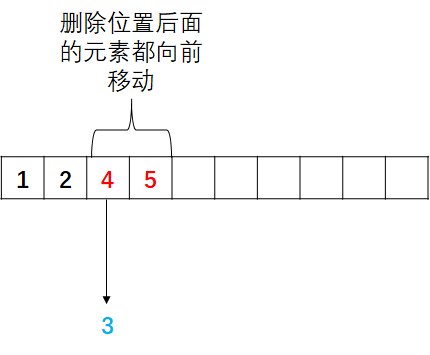1. 判断删除位置pos是否合法
2. 判断顺序表是否为空，若已空，返回-1
3. 将删除位置的元素值进行保存(这里一定要先进行报错，否则移动之后元素值就找不到了)
4. 将pos位置之后的所有元素向前移动一位
5. 顺序表长度length减1，此时删除成功，返回1

int DeleteList(SqList *l,int pos,int *val){
int i;
//判断删除位置是否合法
if(pos < 1 || pos > l->length){
return -1;
}
//判断顺序表是否为空
if(l->length == 0){
return -1;
}
//保存待删除位置的元素值
*val = l->data[pos - 1];
//将pos位置后面的元素向前移动一位
for(i = pos - 1;i <= l->length;i++){
l->data[i] = l->data[i + 1];
}
//顺序表长度减1
l->length--;
return 1;//删除成功
}


1：若删除第一个元素，则需移动n - 1个元素
2：若删除第二个元素，则需移动n - 2个元素
n：依次类推，若删除最后一个元素，则无需移动元素

$\frac{1}{n}[(n - 1)+ (n - 2) + ... + 1 + 0] = \frac{1}{n}\frac{n(n - 1)}{2} = \frac{n - 1}{2}$

## 顺序表的查找

1. 查找指定元素值的位置
2. 查找指定位置的元素值

### 查找指定元素值的位置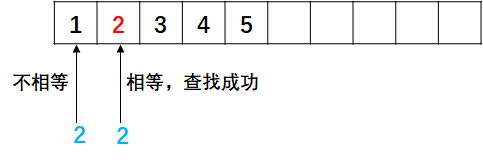int LocateElem(SqList l,int val){
int i;
//若顺序表为空，直接查找失败
if(l.length == 0){
return -1;
}
//循环查找
for(i = 0;i < l.length;i++){
if(l.data[i] == val){
return i + 1;	//返回逻辑位置(下标 + 1)
}
}
return -1;//查找失败
}


• 对于第一个元素1，比较次数为1次
• 对于第二个元素2，比较次数为2次
• 对于第三个元素3，比较次数为3次
• 以此类推，对于第n个元素x，比较次数为n次

$\frac{1}{n}(1 + 2 + 3 + ... + n - 1 + n) = \frac{1}{n}\frac{n(n + 1)}{2} = \frac{n + 1}{2}$

### 查找指定位置的元素值

int GetElem(SqList l,int pos){
//判断pos值的合法性
if(pos < 1 || pos > l.length){
return -1;
}
//判断顺序表是否为空
if(l.length == 0){
return -1;
}
//返回元素值
return l.data[pos - 1];
}


# 源代码

#include <stdio.h>
#include <malloc.h>

#define MAX_SIZE 10		//线性表初始长度
#define ElemType int
typedef struct{
int data[MAX_SIZE];
int length;		//线性表长度

}SqList;

int GetElem(SqList l,int pos){
//判断pos值的合法性
if(pos < 1 || pos > l.length){
return -1;
}
//判断顺序表是否为空
if(l.length == 0){
return -1;
}
//返回元素值
return l.data[pos - 1];
}

int LocateElem(SqList l,int val){
int i;
//若顺序表为空，直接查找失败
if(l.length == 0){
return -1;
}
//循环查找
for(i = 0;i < l.length;i++){
if(l.data[i] == val){
return i + 1;	//返回逻辑位置(下标 + 1)
}
}
return -1;//查找失败
}

int DeleteList(SqList *l,int pos,int *val){
int i;
//判断删除位置是否合法
if(pos < 1 || pos > l->length){
return -1;
}
//判断顺序表是否为空
if(l->length == 0){
return -1;
}
//保存待删除位置的元素值
*val = l->data[pos - 1];
//将pos位置后面的元素向前移动一位
for(i = pos - 1;i <= l->length;i++){
l->data[i] = l->data[i + 1];
}
//顺序表长度减1
l->length--;
return 1;//删除成功
}

int InsertList(SqList *l,int pos,int val){
int i;
//判断插入位置pos是否合法
if(pos < 1 || pos > l->length + 1){
return -1;
}
//判断顺序表是否已满
if(l->length == MAX_SIZE){
return -1;
}
//将pos位置后面的元素向后移动一位
for(i = l->length - 1;i >= pos - 1;i--){
l->data[i + 1] = l->data[i];
}
//将新元素插入到pos位置
l->data[pos - 1] = val;
//顺序表长度加1
l->length++;
return 1;//插入成功
}

SqList InitList(){
//声明顺序表
SqList l;
l.length = 0;
return l;
}

//输出顺序表中元素的函数
void displayTable(SqList l){
int i;
for (i = 0;i < l.length;i++) {
printf("%d\t",l.data[i]);
}
printf("\n");
}


©️2019 CSDN 皮肤主题: 数字20 设计师: CSDN官方博客### 分享到微信朋友圈点击重新获取扫码支付